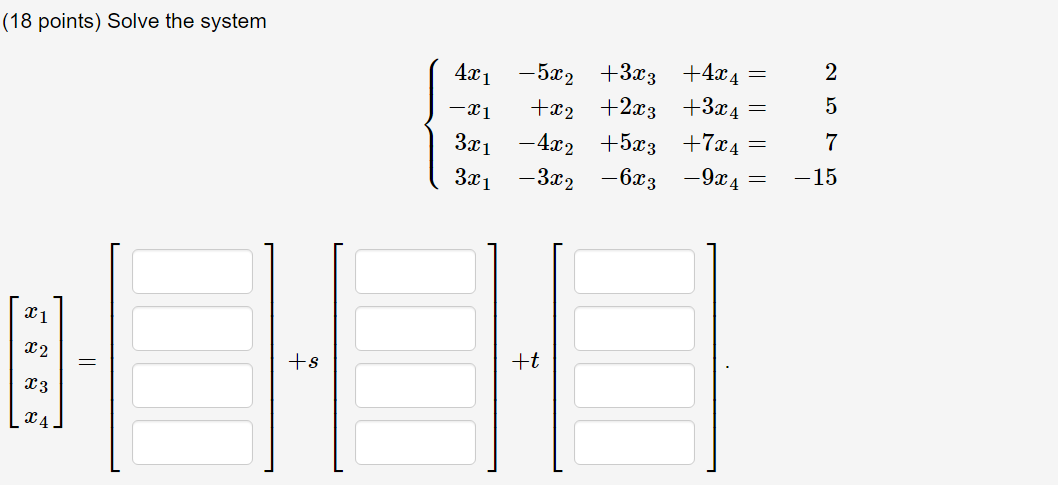💬 👋 We’re always here. Join our Discord to connect with other students 24/7, any time, night or day.Join Here!

MS

Merve S.

Linear Algebra

1 week, 5 days ago

# Could you help me to solve it?UrgentEI

Could you help me to solve it?Urgent

### Discussion

You must be signed in to discuss.

### Video Transcript

mhm Okay and this question we're asked to solve a system and in order to do this I'm going to write this system in matrix form like this and now in order to solve it need to roll reduce it. So first thing that we want to do is to get rid of these elements and we're going to do that by using some row operations. So I'm going to replace route to with four times row two plus one. Row three is going to be four times row 3 -3 times row one. And then roll four is gonna be four times grow 4 -3 times were one. So if you do that you will get This matrix here. So notice that row two equals row three and grow four equals negative three tons road to. So those roads are just going to go away when we reduce them. I'm also gonna multiply road to by negative one to clean it up a little bit. So they'll look like this. Now we want to get rid of this element here and the way to do that is to do girl one equals row one plus five of row two. And that will get you this. Now we can simplify row one By dividing by four. And this is the row reduced form. So When we look at this we read this as one x one plus zero X two minus 13 X three minus 19 X four Equals -27 and zero x 1 Plus one x 2 -11 x 3 -16 X four equals -22. So we can solve X one as 13 X three Plus 19. X 4 -27. We can solve for X two has 11 x three plus 16 X four minus 22. And then replace X three with S. An X four with T. So that will allow us to write X one equals 13 s 1981. X two equals 11. S plus 16. X +41 is 22. And that allows us to write X one X two x 3 X four. As this we have negative 27 plus 13 S. 19 T. We have negative 22 Plus 11 s plus 16 T. This X four should have been A. T. here. Um X three is just one s and zero everything else and then X four is zero plus zero as plus one T. So to get it in the form that's in your problem would write it out like this. There's a T. Out here 1816 01. And that is the solution to this system.

EI
##### Top Linear Algebra Educators##### Lily A.

Johns Hopkins UniversityLectures

Join Bootcamp